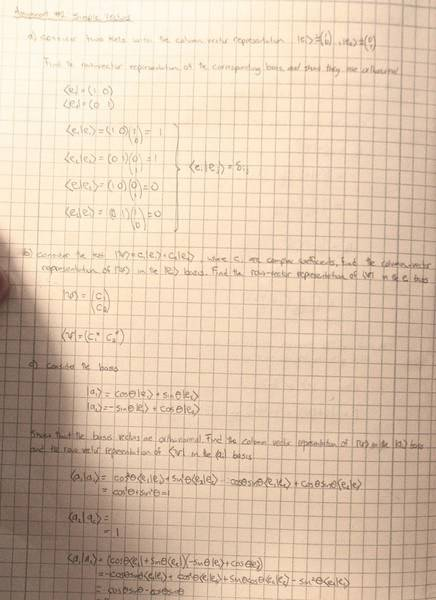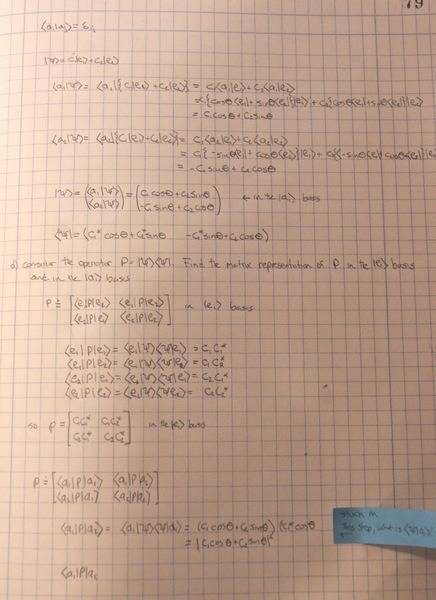# Matrix Representation of Operators

richyw

## Homework Statement

This is quite a long problem, and I have most of it figured out, but I am getting stuck on the very last part of the problem. My problem is I do not understand how to find $\left\langle\psi|a_1\right\rangle$ in the very last line. Is $\left\langle\psi|a_1\right\rangle=(\left\langle a_1|\psi\right\rangle)^*$

## Homework Equations

In a given basis $\left\langle a^{(i)}\right|$, operators are represented by $A_{i,j}=\left\langle a^{(j)}|A|a^{(i)}\right\rangle$

## The Attempt at a SolutionLast edited: